The Theory \ Details \ asset-pricing models \ general approach

# Factor-based Asset Pricing Models

## General approach

Factor-based Asset Pricing Models (asset pricing models for short) help imposing the additional structure on parameters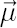andof the analytical model. Restrictions, imposed by an asset pricing model, reduce the number of model parameters that need to be estimated and force “rational” investors to allocate wealth among riskless asset and selected factors only, ignoring the rest of the risky assets. Particular cases of asset pricing models are CAPM and Fama-French 3-Factor Model.

### Linear Regression Equation

Assume that the financial market consists of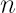financial instruments, first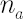of which we denote as assets, the others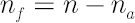we denote as factors1. It is supposed that the dynamics of the market satisfies the conditions of the analytical model withexcess Mu vectorand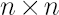covariance matrix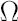. The given parameters can be decomposed according to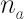assets and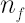factors: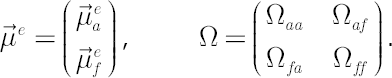Let us denote through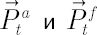vectors of simple rates of return for assets and factors respectively on the interval, where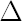is small enough.
Dependence of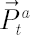from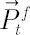can be expressed by the linear regression equation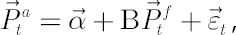where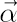is the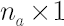vector of mispricing terms (Jensen’s instantaneous alphas);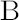is the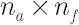matrix of factor sensitivities (Betas);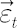is randomvector of residuals with zero averageand such a covariance matrix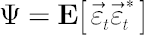that components of vectorsand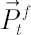are uncorrelated.

The elements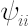of matrix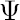are known as residual variances. Their roots are referred to as standard errors of forecast, implied by the regression.

Let us denote sample estimates ofandthrough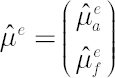and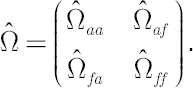Estimates of parametersandare found by means of ordinary least-squares using the following formulas: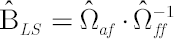### Model Assumptions

The asset pricing model imposes the following restrictions, which must be satisfied by the above regression equation:
1.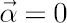.
All mispricing terms in regression equation must be equal to zero.
2.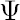is diagonal.
Regression residuals must be uncorrelated.

### Practical Implications

If selected asset pricing model holds true, then the main implication for a CRRA investor (an investor with CRRA utility function) is that there is no sense to invest in individual assets. Corresponding optimal portfolio would contain only riskless asset and portfolio factors as its components. Of course, this is true only when selected factors are available for an investment (i.e. they are at least tradable financial instruments).

### Model-implied Estimates ofandAssuming that the asset pricing model holds true, leads to the modified estimates forand. Model-implied estimatesandare given by the following formulas: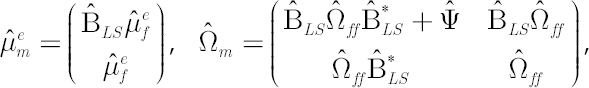where the matrix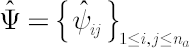is diagonal and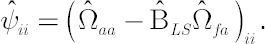### Portfolio Statistics

Portfolio Beta
Consider portfolio. Vectorof portfolio betas can be found in the following way: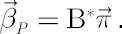Portfolio Variance
Under the selected set of factors the portfolio varianceadmits the following decomposition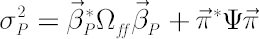into systematic risk (first item) and diversifiable (nonsystematic) risk (second one).

### Other Statistics

Other useful formulae related to asset pricing models include: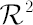Statistics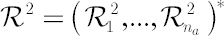is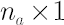vector, whose elements take values in. It is called determination coefficient. The more the valueis closer to unity, the better the selected asset pricing model describes the dynamics of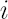-th asset. Calculation formula: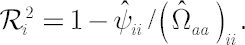Student’s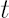-statistics for vectorand matrix-statistics are used in linear regression for testing of hypotheses about regression coefficients beingequal to zero. Formulas for the calculation of-statisticsandare presented below.
Assume thatis the sample size on which the regression coefficients were estimated, and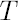is a number of years contained in sample. Then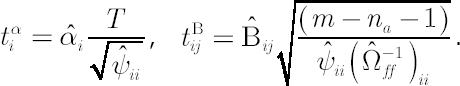The critical two-sided probabilities for Student’s-distribution withdegrees of freedom are obtained based on the absolute values of the calculated statistics. If, then one may use normal distribution instead of-distribution. The values obtained in this way are probabilities of the corresponding regression coefficients being equal to zero.

Note. The following rule of thumb can be proposed: if the absolute value ofstatistics is more than 3, and the number of degrees of freedom is not less than 10, then with high probability (about 99%) the corresponding regression coefficient is significant.

1 Readers shall not confuse the subscript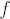used in this section with the riskless rate symbol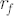.